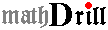Student: guest Logout
 00:00:00
 Score = \n");?> Total Score = \n");?>
If you do not see any problems here in 5 seconds, click on 'More Problems'.Mode : Practice Homework Feedback
 Levels from 1: All Problems 2: Simple 3: Those with Help 4: Ordering+Time 5: Addition 6: Subtraction 7: Multiplication 8: Division 9: Fractions 10: Decimals 11: Word Problems 12: LCM/GCF/RoundUp 13: Algebra/Geometry 14: Percent 15: Basic Addition 16: Basic Subtraction 17: Basic Multiplication 18: Basic Division Select Level 1: Order Numbers 1 2: Order Numbers 2 3: Simple Addition 4: 1 digit Addition 5: 2 digit Addition 6: Word Addition 1 7: Word Addition 2 27: Decimals vs Fractions 1 44: Decimal Multiplication1 53: Linear Eq Word Problems #prob #cols Cursor jump Left Right None